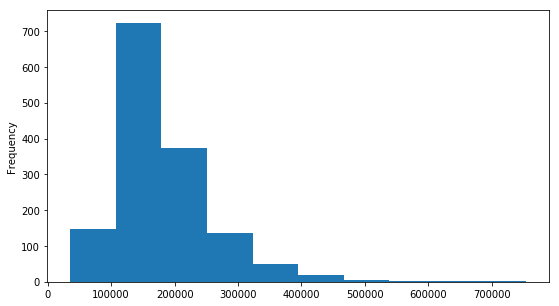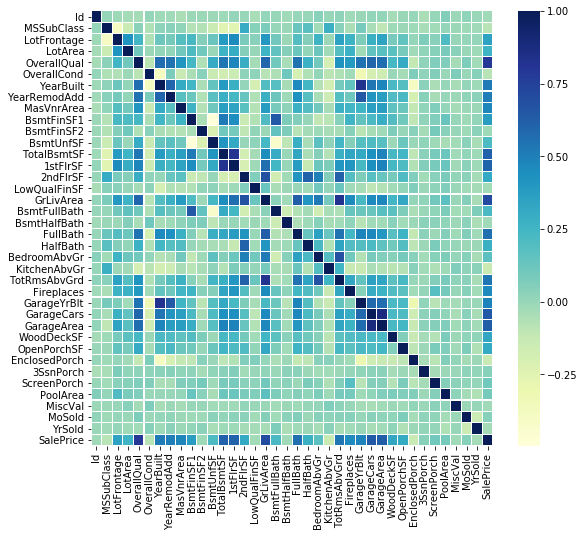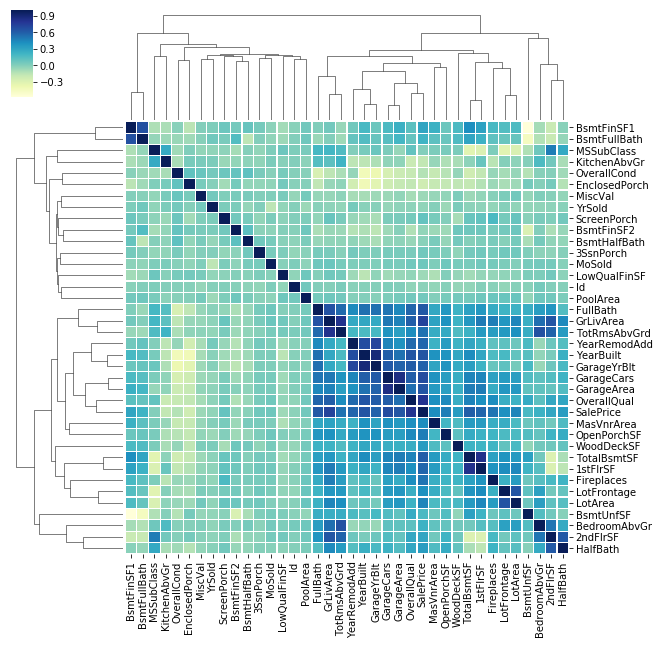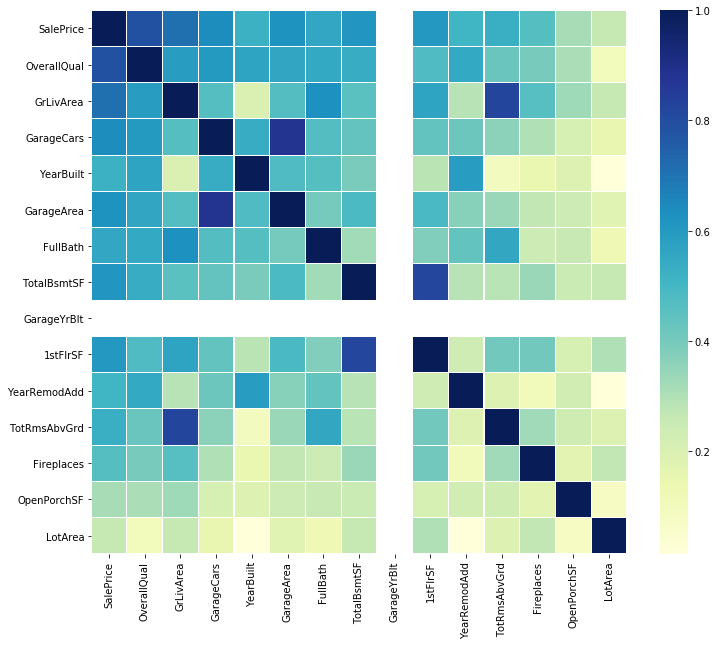Related Articles

# Exploring Correlation in Python

• Last Updated : 19 Jan, 2019

This article aims to give a better understanding of a very important technique of multivariate exploration.

Correlation Matrix is basically a covariance matrix. Also known as the auto-covariance matrix, dispersion matrix, variance matrix, or variance-covariance matrix. It is a matrix in which i-j position defines the correlation between the ith and jth parameter of the given data-set.

When the data points follow a roughly straight-line trend, the variables are said to have an approximately linear relationship. In some cases, the data points fall close to a straight line, but more often there is quite a bit of variability of the points around the straight-line trend. A summary measure called the correlation describes the strength of the linear association. Correlation summarizes the strength and direction of the linear (straight-line) association between two quantitative variables. Denoted by r, it takes values between -1 and +1. A positive value for r indicates a positive association, and a negative value for r indicates a negative association.
The closer r is to 1 the closer the data points fall to a straight line, thus, the linear association is stronger. The closer r is to 0, making the linear association weaker.

 `import` `numpy as np``import` `pandas as pd``import` `seaborn as sns``import` `matplotlib.pyplot as plt``from` `scipy.stats ``import` `norm`

 `data ``=` `pd.read_csv(``"House Price.csv"``)``data.shape`

Output:

`(1460, 81)`

‘Sales Price’ Description

 `data[``'SalePrice'``].describe()`

Output:

```count      1460.000000
mean     180921.195890
std       79442.502883
min       34900.000000
25%      129975.000000
50%      163000.000000
75%      214000.000000
max      755000.000000
Name: SalePrice, dtype: float64```

Histogram

 `plt.figure(figsize ``=` `(``9``, ``5``))``data[``'SalePrice'``].plot(kind ``=``"hist"``)`

Output:Code #1: Correlation Matrix

 `corrmat ``=` `data.corr()`` ` `f, ax ``=` `plt.subplots(figsize ``=``(``9``, ``8``))``sns.heatmap(corrmat, ax ``=` `ax, cmap ``=``"YlGnBu"``, linewidths ``=` `0.1``)`

Output:Code #2: Grid Correlation Matrix

 `corrmat ``=` `data.corr()`` ` `cg ``=` `sns.clustermap(corrmat, cmap ``=``"YlGnBu"``, linewidths ``=` `0.1``);``plt.setp(cg.ax_heatmap.yaxis.get_majorticklabels(), rotation ``=` `0``)`` ` `cg`

Output:Code #3: Correlation for Saleprice

 `# saleprice correlation matrix``# k : number of variables for heatmap``k ``=` `15` ` ` `cols ``=` `corrmat.nlargest(k, ``'SalePrice'``)[``'SalePrice'``].index`` ` `cm ``=` `np.corrcoef(data[cols].values.T)``f, ax ``=` `plt.subplots(figsize ``=``(``12``, ``10``))`` ` `sns.heatmap(cm, ax ``=` `ax, cmap ``=``"YlGnBu"``,``            ``linewidths ``=` `0.1``, yticklabels ``=` `cols.values, ``                              ``xticklabels ``=` `cols.values)`

Output:Attention geek! Strengthen your foundations with the Python Programming Foundation Course and learn the basics.

To begin with, your interview preparations Enhance your Data Structures concepts with the Python DS Course. And to begin with your Machine Learning Journey, join the Machine Learning – Basic Level Course

My Personal Notes arrow_drop_up